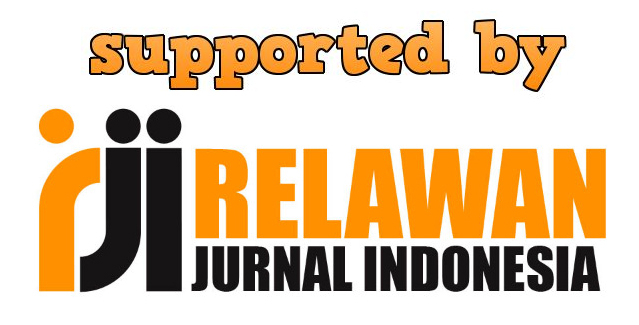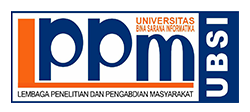### MEMINIMUMKAN BIAYA TRANSPORTASI MENGGUNAKAN METODE BASIS TREE

Maxsi Ary

#### Abstract

Transportation problem is the linear programming problem. The main purpose is minimize the cost of distribution. In present day the solution of transportation problem are North-West Corner, Least Cost, and Vogel’s Approximation (VAM). In 1946-1947 G.B Dantzig proposes the research and designed the MODI (Modified For Distribution), the method could be applied to transportation problem. The MODI method and other method that use a transportation table are adequate as long as the problem is relatively small. Once the problem becomes large, then finding the unique  and performing the updating is difficult. This study was made to find solutions to transportation problems using basis tree. Is a literature study that the authors use to conduct research, with the source form of journal papers, articles and textbooks. The Basis Tree Transportation Problem is a specialization for transportation problem. The key idea in this method is that any basic feasible solution of transportation problem is a spanning tree of the underlying graph. Hence for each iteration, the basis is represent as a rooted spanning tree in which an arc  and its flow  represent the basic variable , and the simplex multiplier (dual variable) are represent by node potential. Use of  Nort-West Corner aimlessly total freight 1490, and the results of the iteration will be obtained a minimum total transportation cost 920. Iterations that use basis tree using five iterations. It can be noted that minimizes transportation costs using the basis tree method resulted in a difference of 570.

Keywords: North-West Corner, Basis Tree, Transportation Problem.

#### Full Text:

PDF

DOI: https://doi.org/10.31294/p.v13i1.3425

ISSN2579-3500